DCP HOME

# Pseudo-Separable L-Convex Minimization

For a finite set V={1,2,...,n} and a family of univariate discrete convex functions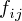andindexed by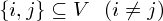and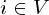, the function defined by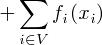is an L-convex function [1,2].

Here we consider the following L-convex functions:

• quadratic pseudo-separable L-convex function: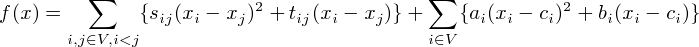• quartic pseudo-separable L-convex function: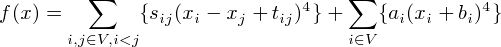• exponential pseudo-separable L-convex function: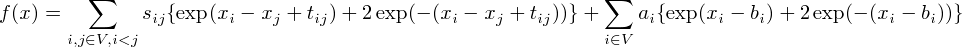• absolute pseudo-separable L-convex function: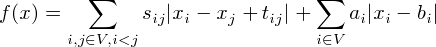This web application minimizes f(x) using ODICON.

 K. Murota (2001): "Discrete Convex Analysis---An Introduction (in Japanese)," Kyoritsu Publishing Company, Tokyo. Section 4.3.

 K. Murota (2003): "Discrete Convex Analysis," SIAM. Section 6.3.

 Satoko Moriguchi(satoko(a)aiit.ac.jp) Replace '(a)' with '@' modified on 5/4 0:05, 2012